Related Articles
Division of Line Segment in Given Ratio – Constructions | Class 10 Maths
• Difficulty Level : Medium
• Last Updated : 05 Nov, 2020

Line is a straight one-dimensional figure that has no thickness. In geometry, a line extends endlessly in both directions. It is described as the shortest distance between any two points. A-line can also be understood as multiple points connected to each other in one specific direction without a gap between them.

Line Segment is a part of a line that is bounded by two different endpoints and contains every point on the line between its endpoints in the shortest possible distance.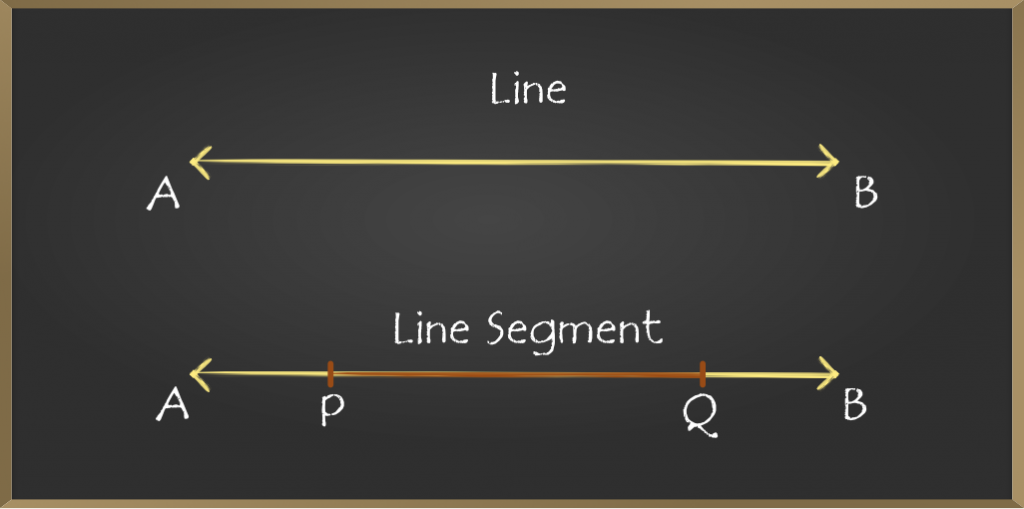### Rules to write down the coordinates of the point which divides the join of two given points P(x1,y1) and Q(x2,y2) internally in a given ratio m1:m2

1. Draw the line segment joining the given points P and Q
2. Write down the coordinates of p and Q at extremities
3. Let R(x, y) be the input which divides PQ internally in the ratio m1:m2
4. For x-coordinate of R, multiply x2 with m1 and x1 with m and add the products. Divide the sum by m1+m2
5. For y-coordinate of R, multiply y2 with m1 and y1 with m2  and add the products. Divide the sum by m1+m2

### Derivation of Formula

Let P(x1, y1) and Q(x2, y2) be the two ends of a given line in a coordinate plane, and R(x,y) be the point on that line which divides PQ in the ratio m1:m2 such that

PR/RQ=m1/m2              …(1)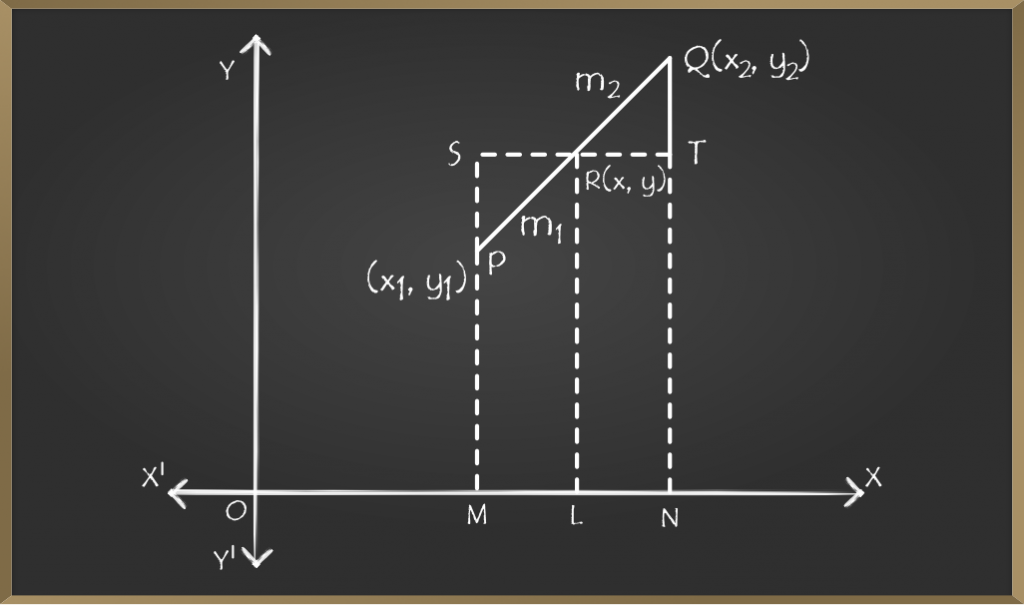Drawing lines PM, QN, and RL perpendicular on the x-axis and through R draw a straight line parallel to the x-axis to meet MP at S and NQ at T.

Hence, from the figure, we can say,

SR = ML = OL – OM = x – x1          …(2)

RT = LN = ON – Ol = x2 – x            …(3)

PS = MS – MP = LR – MP = y – y1    …(4)

TQ = NQ – NT = NQ – LR = y2 – y   …(5)

Now SPR is similar to TQR

SR / RT = PR / RQ

By using equation 2, 3, and 1, we know,

x – x1 / x2 – x = m1 / m2

m2x – m2x1 = m1x2 – m1x

m1x + m2x = m1x2 + m2x1

(m1 + m2)x = m1x2 + m2x1

x = (m1x2 + m2x1) / (m1 + m2)

Now SPR is similar to TQR,

Therefore,

PS/TQ = PR/RQ

By using equation 4, 5, and 1, we know,

y – y1 / y2 – y = m1/m2

m2y – m2y1 = m1y2 – m1y

m1y + m2y = m1y2 + m2y1

(m1 + m2)y = m1y2 + m2y1

y = (m1y2 + m2y1)/(m1 + m2)

Hence, the coordinate of R(x,y) are,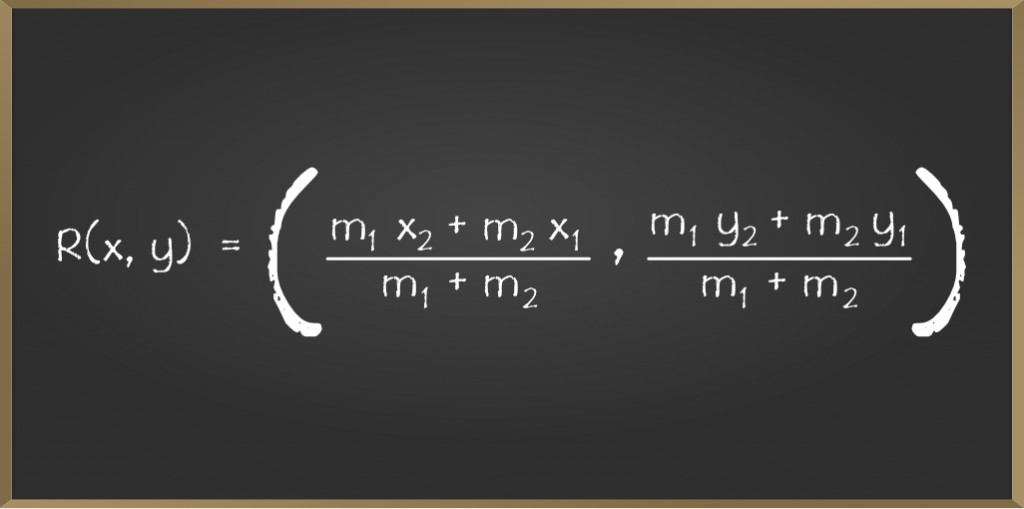### Sample Problems based on the Formula

Problem 1: Calculate the co-ordinates of the point P which divides the line joining A(-3,3) and B(2,-7) in the ratio 2:3

Solution:

Let (x, y) be th co-ordinates of the point P which divides the line joining A(-3, 3) and B(2, -7) in the ratio 2:3, then

Applying the formulae,

x = (m1x2 + m2x1) / (m1 + m2)

x = {2 x 2 + 3 x (-3)} / (2 + 3)

x = (4 – 9) / 5

x = -5 / 5

x = -1

y = (m1y2 + m2y1) / (m1 + m2)

y = {2 x (-7) + 3 x 3} / (2 + 3)

y = (-14 + 9) / 5

y = -5 / 5

y = -1

Hence the co-ordinates of point P are (-1, -1).

Problem 2: If the line joining the points A(4,-5) and B(4,5) is divided by point P such that AP/AB=2/5, find the coordinates of P.

Solution:

Given AP/AB = 2/5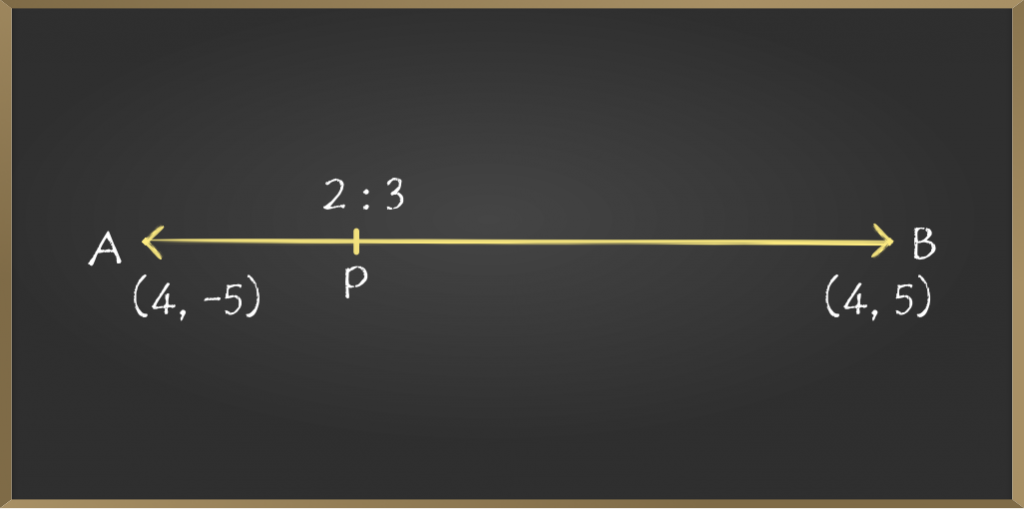5AP = 2AB = 2(AP + PB)

3AP = 2PB

AP/PB = 2/3

AP:PB = 2:3

Thus the point P divides the line segment joining the points A(4, -5) and B(4, 5) in the ratio 2:3 internally.

Hence the coordinates of P are,

Px = (2 x 4 + 3 x 4)/(2 + 3)

= (8 + 12)/5

= 20/5

= 4

Py = (2 x 5 + 3 x (-5))/(2 + 3)

= (10 – 15)/5

= -5/5

= -1

Coordinates of P are (4, -1).

Problem 3: In what ratio does the point P(2, -5) divide the line segment joining the points A(-3, 5) and B(4, -9).

Solution:

Let P(2, -5) divide the line segment joining the points A(-3, 3) and B(4, -9) in the ratio k:1, i.e. AP:PB = k:1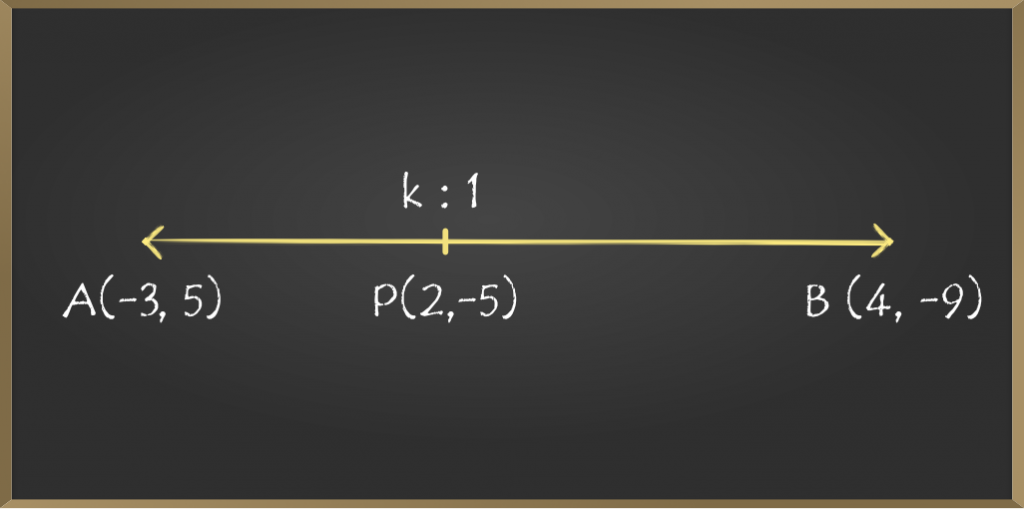Therefore, Coordinates of P are,

Px = (k.4 + 1.(-3)) / (k + 1)

Py = (k.(-9) + 1 x 5)/(k + 1)

But P is (2, -5) therefore,

(4k – 3) / (k + 1) = 2 and (-9k + 5) / (k + 1) = -5

Solving any of the two equation we get k = 5/2

Therefore, the required ratio is 5/2:1 i.e. 5:2(internally)

Attention reader! Don’t stop learning now. Get hold of all the important DSA concepts with the DSA Self Paced Course at a student-friendly price and become industry ready.

My Personal Notes arrow_drop_up